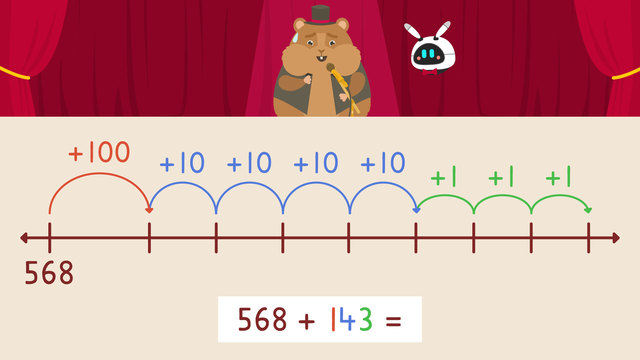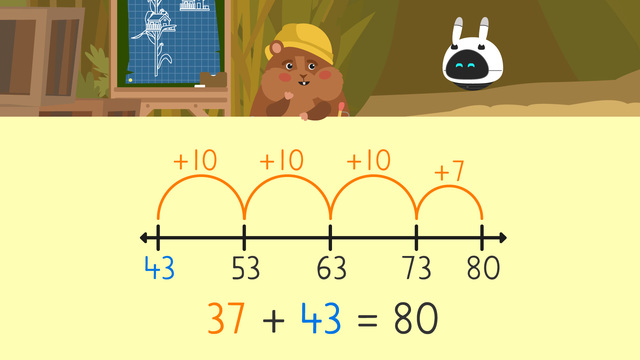# Three Digit Addition with Regrouping - Number LineRating

Ø 5.0 / 1 ratings
The authorsTeam Digital
Three Digit Addition with Regrouping - Number Line
CCSS.MATH.CONTENT.3.NBT.A.2

## 3 Digit Addition with Regrouping on Number Lines

Addition and subtraction are taught in math in the early stages. We are familiar with the addition or subtraction process from real life examples. Also, we do some mathematical operations like addition or subtraction at least a couple of times a day. In this learning text, we will show addition using the number line to add 3-digit numbers. This text will explain how number lines can visualize three-digit addition with regrouping. You can refresh your memory on how to create number lines for additions.## Three Digit Addition with Regrouping on a Number Line – Explanation

The number line can help us to model an equation. In our examples, we use an open number line to show the movement while adding different amounts.

We are going to add two numbers: 306 and 262.

• Firstly, draw a number line and plot the larger number on the left-hand side of the drawn number line.
• Secondly, write the amount you are adding in expanded form.Then, start adding starting with hundreds, jump by hundreds twice and plot the answer.Now move to the tens and start jumping by tens six times and write the answer.Finally, move to the ones and skip forward twice and put the final answer.Our solution from the addition of adding 306 to 262 is 568.

## Three Digit Addition with Regrouping on a Number Line – Practice Example

We are going to show another example of 3-digit addition with a regrouping of the open number line. This time we are going to add 568 and 143. We are going to follow the same steps:

• Firstly, draw a number line and plot the larger number on the left-hand side of the drawn number line.
• Secondly, write the amount you are adding in expanded form• Then, start adding beginning with hundreds and jump by hundred once and plot the answer.
• Then, move to the tens and start jumping by tens four times and write the answer.
• Finally, move to the ones and jump forward three times and put the final answer.Our solution from the addition of 568 and 143 is 711.

## Three Digit Addition with Regrouping on Number Lines – Summary

To solve addition equations with regrouping we can model with an open number line and follow the steps:

Step # What to Do
1 Draw a number line and plot the larger lumber on the left-hand side.
2 Write the amount you are adding in expanded form.
3 Skip count forward by the hundreds, tens, and ones, plotting the number as you go.
4 The final number is the solution.

Now you should be able to show addition using the number line to add 3-digit numbers. Feel free to watch the video explaining the addition using an open number line to add 3-digit numbers, or complete the worksheet.